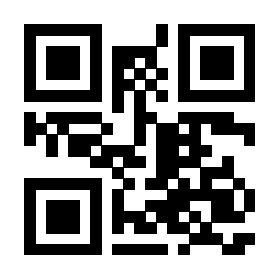# 如何利用 codehigh 宏包的 demohigh 环境插入自定义宏的抄录代码

0

179

\documentclass{article}
\usepackage{codehigh}
\usepackage[margin=1cm]{geometry}
\begin{document}
\makeatletter
\begin{demohigh}
%\makeatletter
\def\@cmd[#1]#2{\textcolor{red}{#1} and (#2)}
\def\cmd{
\@ifnextchar[{\@cmd}{\@cmd[default]}
}
\cmd{aaa}\par
\cmd[bbb]{aaa}\par

\def\@cmd[#1]#2{\c@md{} and \textcolor{red}{#1} and (#2)}
\def\cmd#1{
\def\c@md{#1}\@ifnextchar[{\@cmd}{\@cmd[default]}
}
\cmd{aaa}{ccc}\par
\cmd{aaa}[bbb]{ccc}
%\makeatother

\end{demohigh}
\makeatother
\end{document}

win11 + texlive2022 + xelatex && pdflatex3 个回答

### 撰写答案